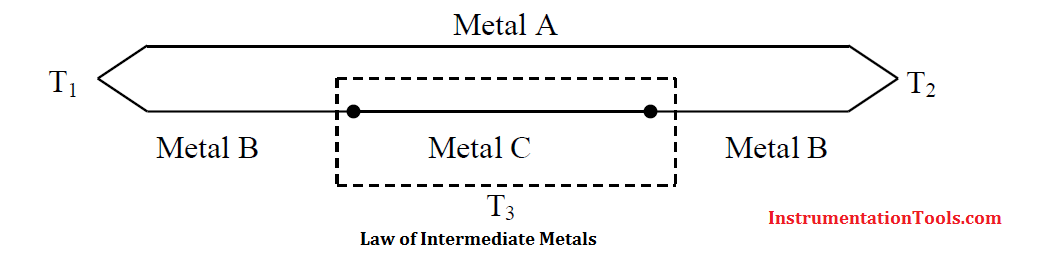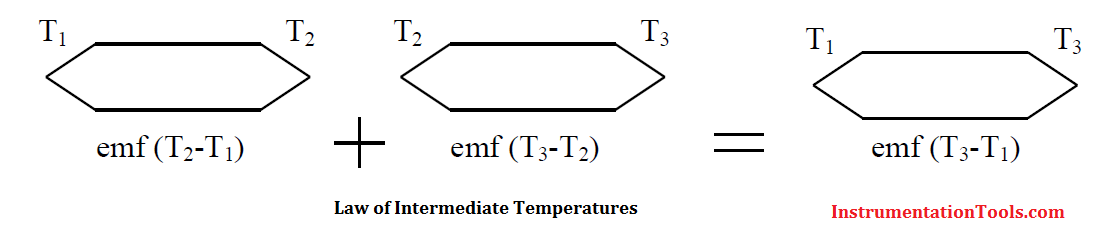# Laws of Thermocouples

The following empirically derived thermocouple laws, are useful to understand, diagnose and utilise thermocouples.

a) Law of homogeneous circuits

If two thermocouple junctions are at T1 and T2, then the thermal emf generated is independent and unaffected by any temperature distribution along the wires.In above Figure, a thermocouple is shown with junction temperatures at T1 and T2. Along the thermocouple wires, the temperature is T3 and T4. The thermocouple emf is, however, still a function of only the temperature gradient T2 – T1.

b) Law of intermediate metals

The law of intermediate metals states that a third metal may be inserted into a thermocouple system without affecting the emf generated, if, and only if, the junctions with the third metal are kept at the same temperature.When thermocouples are used, it is usually necessary to introduce additional metals into the circuit This happens when an instrument is used to measure the emf, and when the junction is soldered or welded. It would seem that the introduction of other metals would modify the emf developed by the thermocouple and destroy its calibration. However, the law of intermediate metals states that the introduction of a third metal into the circuit will have no effect upon the emf generated so long as the junctions of the third metal are at the same temperature, as shown in Above Figure

c) Law of intermediate temperatures

The law of intermediate temperatures states that the sum of the emf developed by a thermocouple with its junctions at temperatures T1 and T2, and with its junctions at temperatures T2 and T3, will be the same as the emf developed if the thermocouple junctions are at temperatures T1 and T3.This law, illustrated in above Figure, is useful in practice because it helps in giving a suitable correction in case a reference junction temperature other than 0 °C is employed. For example, if a thermocouple is calibrated for a reference junction temperature of 0 °C and used with a junction temperature of 20 °C, then the correction required for the observation would be the emf produced by the thermocouple between 0 °C and 20 °C.

Also Read: RTD or Thermocouple ?